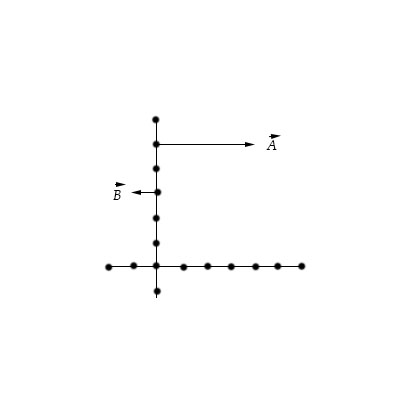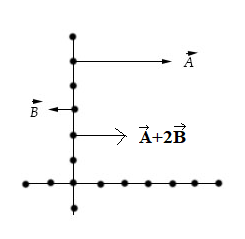# Draw the vector \vec C = \vec A + 2 \vec B.

## Question:

Draw the vector {eq}\vec C = \vec A + 2 \vec B. {/eq}## Sum of Vector:

A vector is an element of a vector space that is described by three basic information: a modulus (length), a direction and verse.

The addition of two vectors can be found by applying the parallelogram rule. To this end, the vectors are placed head to tail and

the sum is equal to the vector from the free tail to the free head.

The sum of the vectors in the figure reported below is found by applying the parallelogram rule. To this end, the vectors are placed head to tail and

the sum is equal to the vector from the free tail to the free head.

The vector sum {eq}\vec C = \vec A + 2 \vec B. {/eq} is reported in the figure below.